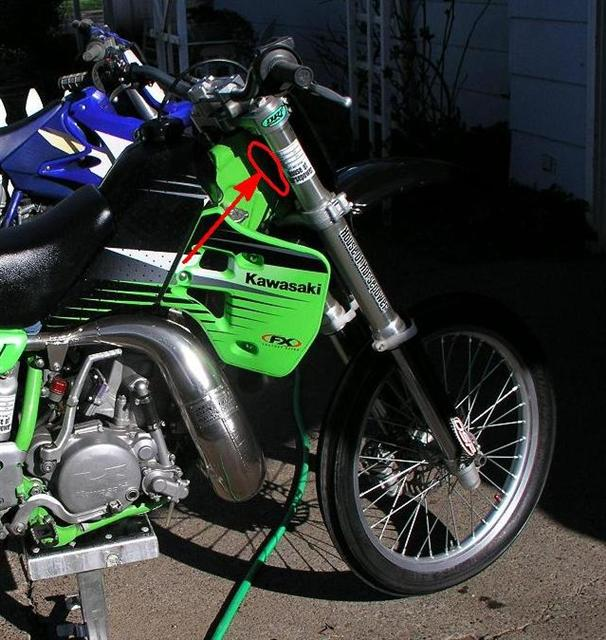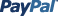The northwest premiere used dirt bike parts store. Quality vintage, motocross, dual sport and modern used dirt bike parts. Your dirt bike engine and part information source.

What year is my dirt bike?

The frame is easy.

Find the VIN number stamped on your frame.

The VIN is located on the front part of your bike just below the bars.COUNT FROM LEFT TO RIGHT 10 CHARACTERS. Compare the 10th character of your VIN with the chart below.

If your 10th digit is a "V", look at the chart and find "V".

If your10th digit is a "3", look at the chart and find "3".

 1980 = A 1981 = B 1982 = C 1983 = D 1984 = E 1985 = F 1986 = G 1987 = H 1988 = J 1989 = K 1990 = L 1991 = M 1992 = N 1993 = P 1994 = R 1995 = S 1996 = T 1997 = V 1998 = W 1999 = X 2000 = Y 2001 = 1 2002 = 2 2003 = 3 2004 = 4 2005 = 5 2006 = 6 2007 = 7 2008 = 8 2009 = 9 2010 = A 2011 = B 2012 = C 2013 = D 2014 = E 2015 = F 2016 = G 2017 = H 2018 = J 2019 = K 2020 = L 2021 = M

So now we have answered your question "What year is my dirt bike?"

At least now you know what year your frame is.

How do you know if your engine is the same year as your frame?